Next: Lagrangian Dynamics Up: Rigid Body Rotation Previous: Rotational Stability

Exercises

1. Find the principal axes of rotation and the principal moments of inertia for a thin uniform rectangular plate of mass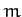and dimensions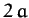by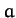for rotation about axes passing through (a) the center of mass, and (b) a corner.

2. A rigid body having an axis of symmetry rotates freely about a fixed point under no torques. Ifis the angle between the symmetry axis and the instantaneous axis of rotation, show that the angle between the axis of rotation and the invariable line (the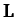vector) is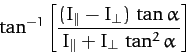where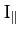(the moment of inertia about the symmetry axis) is greater than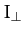(the moment of inertia about an axis normal to the symmetry axis).

3. Since the greatest value of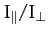is 2 (symmetrical lamina) show from the previous result that the angle between the angular velocity and angular momentum vectors cannot exceed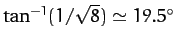. Find the corresponding value of.

4. A thin uniform rod of lengthand massis constrained to rotate with constant angular velocity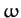about an axis passing through the center of the rod, and making an anglewith the rod. Show that the angular momentum about the center of the rod is perpendicular to the rod, and is of magnitude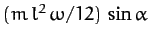. Show that the torque is perpendicular to both the rod and the angular momentum vector, and is of magnitude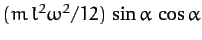.

5. A thin uniform disk of radiusand massis constrained to rotate with constant angular velocityabout an axis passing through its center, and making an anglewith the normal to the disk. Find the angular momentum about the center of the disk, as well as the torque acting on the disk.

6. Demonstrate that for an isolated rigid body which possesses an axis of symmetry, and rotates about one of its principal axes, the motion is only stable to small perturbations if the principal axis is that which corresponds to the symmetry axis.Next: Lagrangian Dynamics Up: Rigid Body Rotation Previous: Rotational Stability
Richard Fitzpatrick 2011-03-31